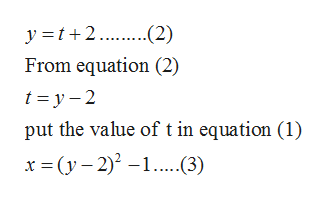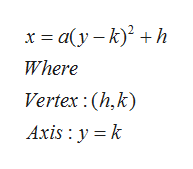# Graph the parametric graph and find the corresponding rectangular equation by eliminating the parameter. x=t^2-1,y=t+2.

Question
4 views

Graph the parametric graph and find the corresponding rectangular equation by eliminating the parameter. x=t^2-1,y=t+2.

check_circle

Step 1

Given curve is

Step 2

The corresponding rectangular equation by eliminating the parameter ishelp_outlineImage Transcriptionclosey =t+2. .(2) From equation (2) t = y – 2 put the value of t in equation (1) x = (y – 2) –1..(3) fullscreen
Step 3

Here, equation (3) represents the equation of parabo...help_outlineImage Transcriptionclosex = a(y – k)? + h Where Vertex : (h,k) Axis : y = k fullscreen

### Want to see the full answer?

See Solution

#### Want to see this answer and more?

Solutions are written by subject experts who are available 24/7. Questions are typically answered within 1 hour.*

See Solution
*Response times may vary by subject and question.
Tagged in

### Calculus